# Chapter 10 And Finally The Stack Stacks A

• Slides: 19Chapter 10 And, Finally. . . The Stack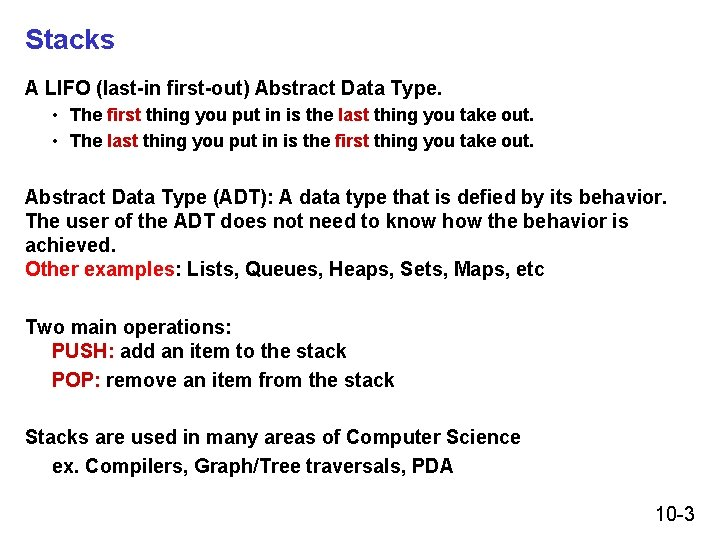Stacks A LIFO (last-in first-out) Abstract Data Type. • The first thing you put in is the last thing you take out. • The last thing you put in is the first thing you take out. Abstract Data Type (ADT): A data type that is defied by its behavior. The user of the ADT does not need to know how the behavior is achieved. Other examples: Lists, Queues, Heaps, Sets, Maps, etc Two main operations: PUSH: add an item to the stack POP: remove an item from the stack Stacks are used in many areas of Computer Science ex. Compilers, Graph/Tree traversals, PDA 10 -3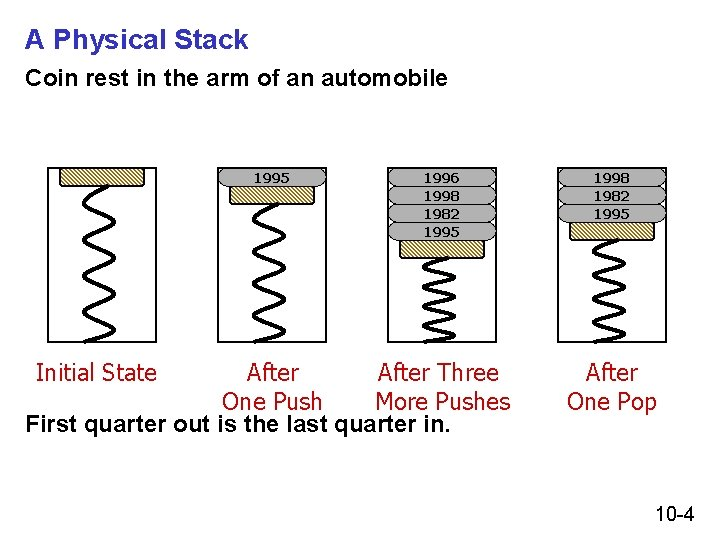A Physical Stack Coin rest in the arm of an automobile 1995 Initial State 1996 1998 1982 1995 After Three One Push More Pushes First quarter out is the last quarter in. 1998 1982 1995 After One Pop 10 -4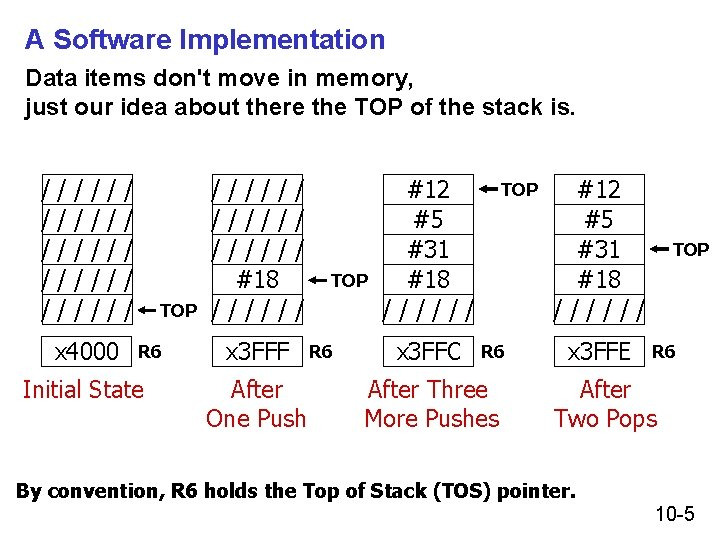A Software Implementation Data items don't move in memory, just our idea about there the TOP of the stack is. / / / / / / / x 4000 / / / TOP R 6 Initial State ///// #18 ///// / TOP / x 3 FFF After One Push R 6 #12 #5 #31 #18 ////// x 3 FFC TOP R 6 After Three More Pushes #12 #5 #31 #18 ////// x 3 FFE TOP R 6 After Two Pops By convention, R 6 holds the Top of Stack (TOS) pointer. 10 -5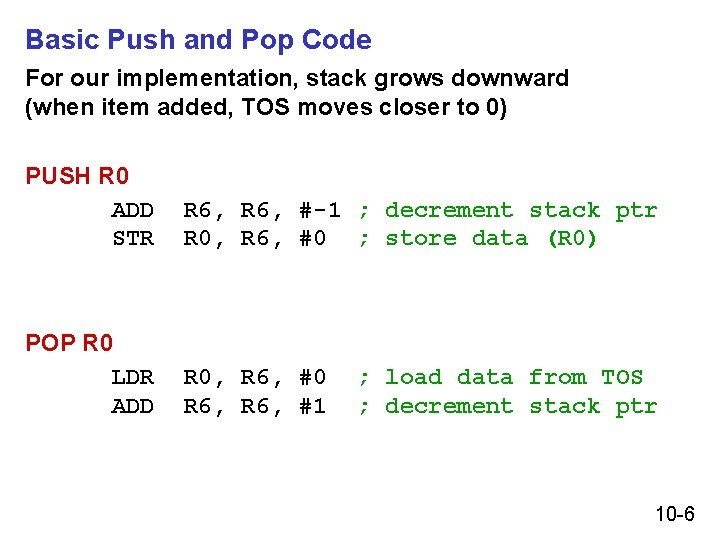Basic Push and Pop Code For our implementation, stack grows downward (when item added, TOS moves closer to 0) PUSH R 0 ADD STR R 6, #-1 ; decrement stack ptr R 0, R 6, #0 ; store data (R 0) POP R 0 LDR ADD R 0, R 6, #0 R 6, #1 ; load data from TOS ; decrement stack ptr 10 -6Pop with Underflow Detection If we try to pop too many items off the stack, an underflow condition occurs. • Check for underflow by checking TOS before removing data. • Return status code in R 5 (0 for success, 1 for underflow) POP LD R 1, EMPTY ADD R 2, R 6, R 1 BRzp FAIL LDR R 0, R 6, #0 ADD R 6, #1 AND R 5, #0 RET FAIL AND R 5, #0 ADD R 5, #1 RET EMPTY. FILL x. C 000 ; EMPTY = -x 4000 ; Compare stack pointer ; ; SUCCESS: R 5 = 0 ; FAIL: R 5 = 1 10 -7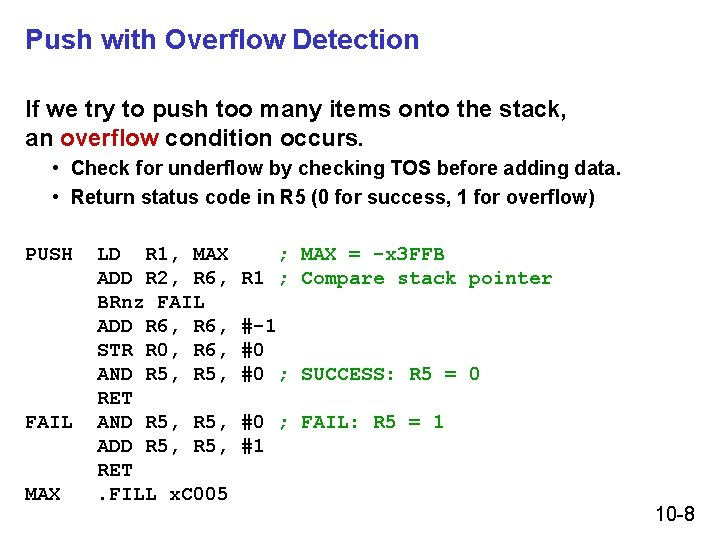Push with Overflow Detection If we try to push too many items onto the stack, an overflow condition occurs. • Check for underflow by checking TOS before adding data. • Return status code in R 5 (0 for success, 1 for overflow) PUSH FAIL MAX LD R 1, MAX ADD R 2, R 6, BRnz FAIL ADD R 6, STR R 0, R 6, AND R 5, RET AND R 5, ADD R 5, RET. FILL x. C 005 ; MAX = -x 3 FFB R 1 ; Compare stack pointer #-1 #0 #0 ; SUCCESS: R 5 = 0 #0 ; FAIL: R 5 = 1 #1 10 -8Data Type Conversion These routines in the following slides might be useful. 10 -9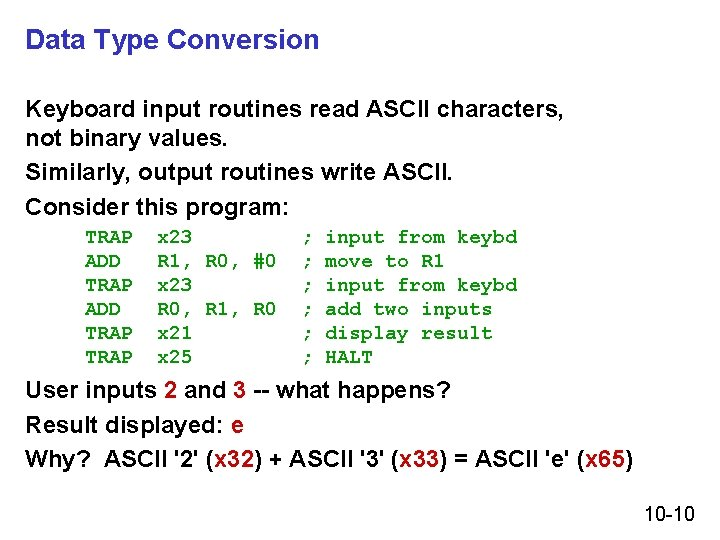Data Type Conversion Keyboard input routines read ASCII characters, not binary values. Similarly, output routines write ASCII. Consider this program: TRAP ADD TRAP x 23 R 1, R 0, #0 x 23 R 0, R 1, R 0 x 21 x 25 ; ; ; input from keybd move to R 1 input from keybd add two inputs display result HALT User inputs 2 and 3 -- what happens? Result displayed: e Why? ASCII '2' (x 32) + ASCII '3' (x 33) = ASCII 'e' (x 65) 10 -10ASCII to Binary Useful to deal with mult-digit decimal numbers Assume we've read three ASCII digits (e. g. , "259") into a memory buffer. How do we convert this to a number we can use? • Convert first character to digit (subtract x 30) and multiply by 100. • Convert second character to digit and multiply by 10. • Convert third character to digit. • Add the three digits together. x 32 x 35 x 39 '2' '5' '9' 10 -11Multiplication via a Lookup Table How can we multiply a number by 100? • One approach: Add number to itself 100 times. • Another approach: Add 100 to itself <number> times. (Better if number < 100. ) Since we have a small range of numbers (0 -9), use number as an index into a lookup table. Entry 0: Entry 1: Entry 2: Entry 3: etc. 0 x 100 = 0 1 x 100 = 100 2 x 100 = 200 3 x 100 = 300 10 -12Code for Lookup Table ; multiply R 0 by 100, using lookup table ; LEA R 1, Lookup 100 ; R 1 = table base ADD R 1, R 0 ; add index (R 0) LDR R 0, R 1, #0 ; load from M[R 1]. . . Lookup 100. FILL 0 ; entry 0. FILL 100 ; entry 1. FILL 200 ; entry 2. FILL 300 ; entry 3. FILL 400 ; entry 4. FILL 500 ; entry 5. FILL 600 ; entry 6. FILL 700 ; entry 7. FILL 800 ; entry 8. FILL 900 ; entry 9 10 -13Complete Conversion Routine (1 of 3) ; Three-digit buffer at ASCIIBUF. ; R 1 tells how many digits to convert. ; Put resulting decimal number in R 0. ASCIIto. Binary AND R 0, #0 ; clear result ADD R 1, #0 ; test # digits BRz Done. Ato. B ; done if no digits ; LD R 3, Neg. Zero ; R 3 = -x 30 LEA R 2, ASCIIBUF ADD R 2, R 1 ADD R 2, #-1 ; points to ones digit ; LDR R 4, R 2, #0 ; load digit ADD R 4, R 3 ; convert to number ADD R 0, R 4 ; add ones contrib 10 -14Conversion Routine (2 of 3) ADD BRz ADD R 1, #-1 Done. Ato. B R 2, #-1 ; one less digit ; done if zero ; points to tens digit LDR ADD LEA ADD LDR ADD R 4, R 5, R 4, R 0, ; load digit ; convert to number ; multiply by 10 ADD BRz ADD R 1, #-1 Done. Ato. B R 2, #-1 ; R 2, #0 R 4, R 3 Lookup 10 R 5, R 4 R 5, #0 R 0, R 4 ; adds tens contrib ; ; ; one less digit done if zero points to hundreds digit 10 -15Conversion Routine (3 of 3) LDR ADD LEA ADD LDR ADD ; Done. Ato. B Neg. Zero ASCIIBUF Lookup 10. . . Lookup 100 RET. FILL. BLKW. FILL R 4, R 5, R 4, R 0, R 2, #0 ; load digit R 4, R 3 ; convert to number Lookup 100 ; multiply by 100 R 5, R 4 R 5, #0 R 0, R 4 ; adds 100's contrib x. FFD 0 4 0 10 20 ; -x 30 . FILL 0. FILL 100 . . . 10 -16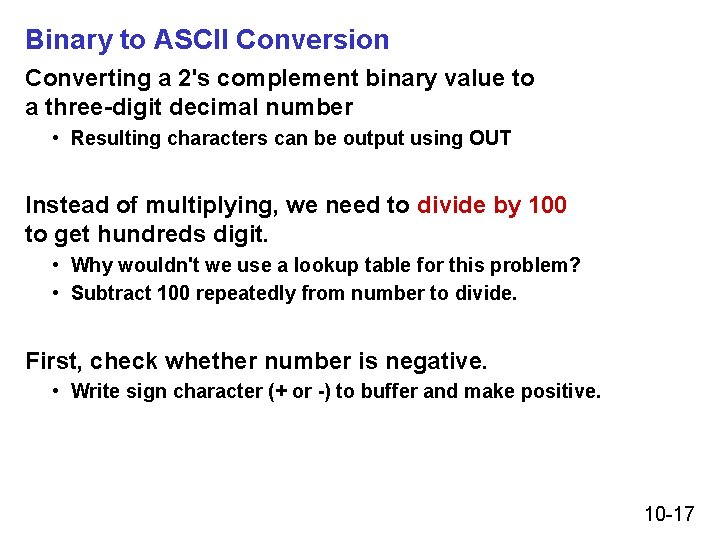Binary to ASCII Conversion Converting a 2's complement binary value to a three-digit decimal number • Resulting characters can be output using OUT Instead of multiplying, we need to divide by 100 to get hundreds digit. • Why wouldn't we use a lookup table for this problem? • Subtract 100 repeatedly from number to divide. First, check whether number is negative. • Write sign character (+ or -) to buffer and make positive. 10 -17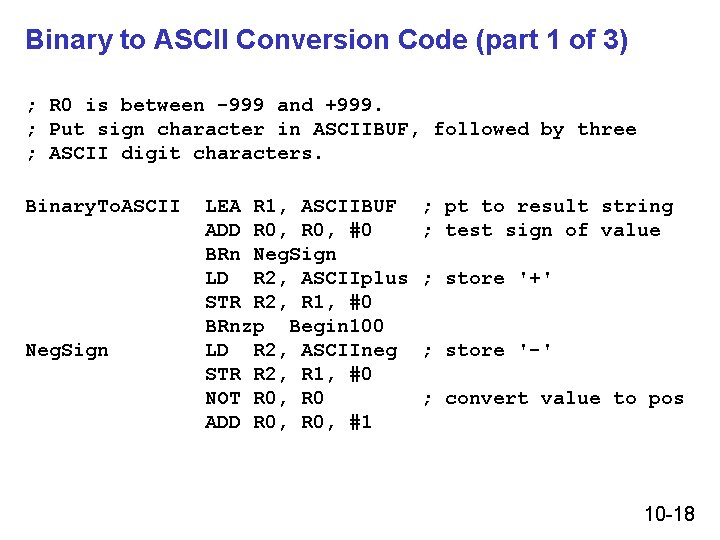Binary to ASCII Conversion Code (part 1 of 3) ; R 0 is between -999 and +999. ; Put sign character in ASCIIBUF, followed by three ; ASCII digit characters. Binary. To. ASCII Neg. Sign LEA R 1, ASCIIBUF ADD R 0, #0 BRn Neg. Sign LD R 2, ASCIIplus STR R 2, R 1, #0 BRnzp Begin 100 LD R 2, ASCIIneg STR R 2, R 1, #0 NOT R 0, R 0 ADD R 0, #1 ; pt to result string ; test sign of value ; store '+' ; store '-' ; convert value to pos 10 -18Conversion (2 of 3) Begin 100 Loop 100 End 100 LD R 2, ASCIIoffset LD R 3, Neg 100 ADD R 0, R 3 BRn End 100 ADD R 2, #1 ; add one to digit BRnzp Loop 100 STR R 2, R 1, #1 ; store ASCII 100's digit LD R 3, Pos 100 ADD R 0, R 3 ; restore last subtract ; Loop 100 LD R 2, ASCIIoffset LD R 3, Neg 10 ADD R 0, R 3 BRn End 10 ADD R 2, #1 ; add one to digit BRnzp Loop 10 10 -19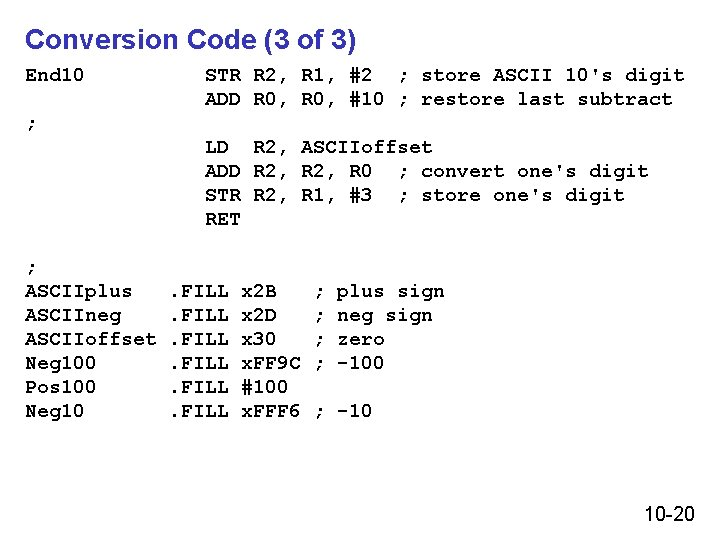Conversion Code (3 of 3) End 10 STR R 2, R 1, #2 ; store ASCII 10's digit ADD R 0, #10 ; restore last subtract ; LD R 2, ASCIIoffset ADD R 2, R 0 ; convert one's digit STR R 2, R 1, #3 ; store one's digit RET ; ASCIIplus ASCIIneg ASCIIoffset Neg 100 Pos 100 Neg 10 . FILL x 2 B x 2 D x 30 x. FF 9 C #100 x. FFF 6 ; ; plus sign neg sign zero -100 ; -10 10 -20